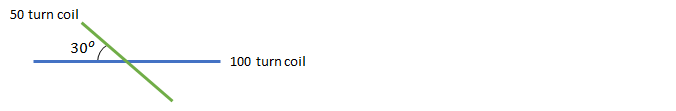Problem: A circular coil with 50 turns and a radius of 2 cm lies at the center of a larger coil with 100 turns and a radius of 5 cm. While the center of each loop coincides, the smaller loop's surface is rotated 30° away from the surface of the larger loop, as shown in the figure (from a top-down perspective). If the larger loop carries a current of 10 A, and the smaller loop carries a current of 15 A in the same direction, what is the magnitude of the magnetic field at the center of the loops?

FREE Expert Solution
93% (453 ratings)
Problem Details

A circular coil with 50 turns and a radius of 2 cm lies at the center of a larger coil with 100 turns and a radius of 5 cm. While the center of each loop coincides, the smaller loop's surface is rotated 30° away from the surface of the larger loop, as shown in the figure (from a top-down perspective). If the larger loop carries a current of 10 A, and the smaller loop carries a current of 15 A in the same direction, what is the magnitude of the magnetic field at the center of the loops?